# Filaments

Posted on in Code Sketches

Tags: processing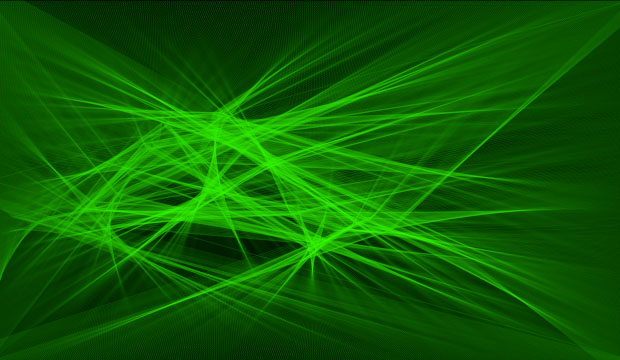Recently I read about, and then watched, Casey Reas’ Process Compendium 2004-2010 on Creative Applications Network. I loved the way he characterized a generative system:

An Element is a simple machine that is comprised of a Form and one or more Behaviors. A Process defines an environment for Elements and determines how the relationships between the Elements are visualized.

https://reas.com/compendium_text/

The above sketch could be described in this paradigm as follows:

Forms
F1: Point
F2: Line

Behaviors
B1: Move horizontally across the screen
B2: Move vertically according to a sin function
B3: Adjust speed and position according to a noise function
B4: Move along an edge of the screen

Elements
E1: F1 + B1 + B2 + B3
E2: F1 + B4
E3: E1 + E2

Screen captures and source code below… most of the parameters are at the top of the code and fun to play with, so please feel free to experiment, and leave me a comment if you do. Made with Processing.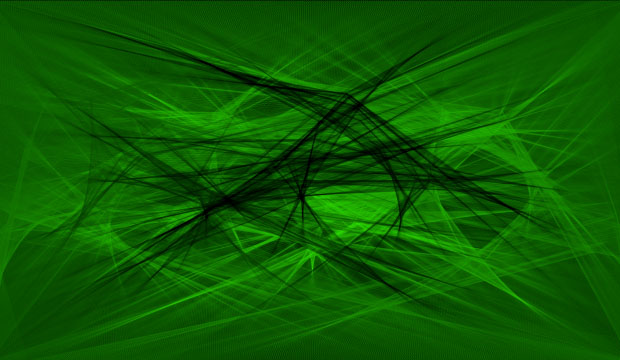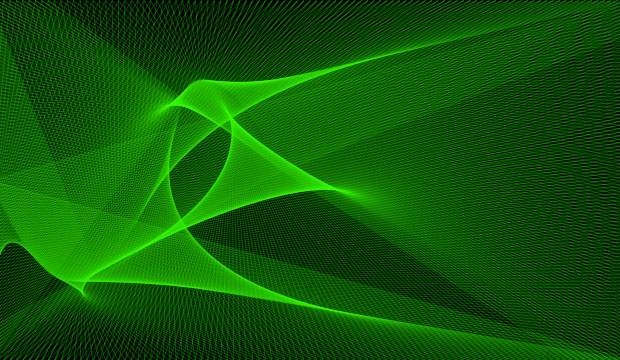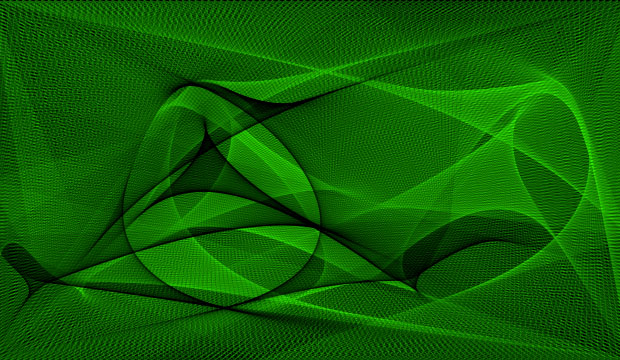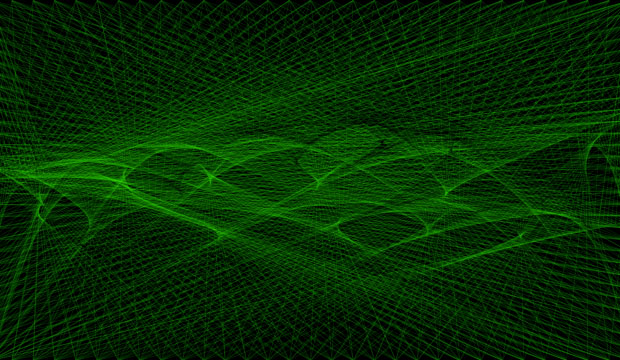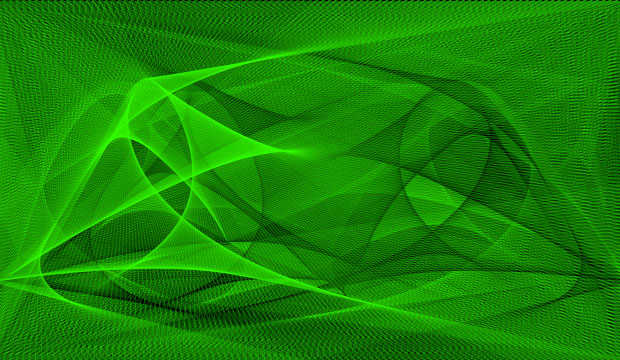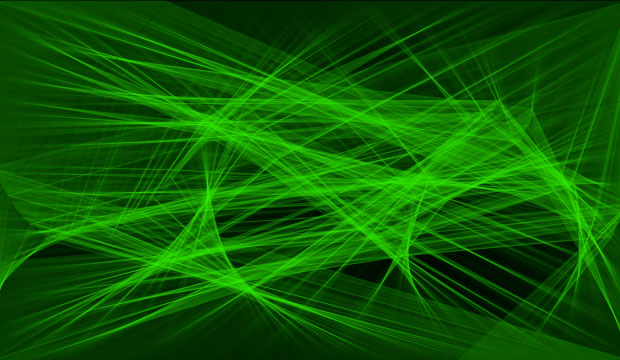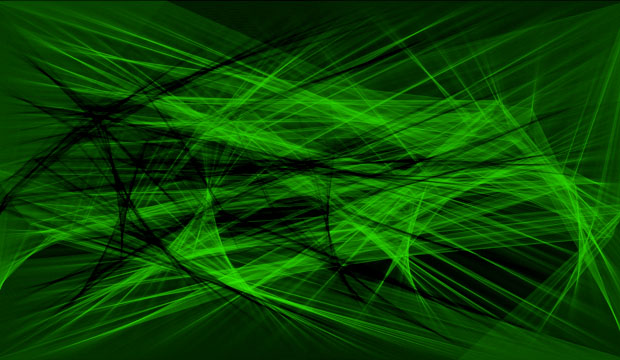``````// Processing code
PVector pt, pt0, pt1, pt2, pt3;
float speed = 1,
noiseScale = 100, // higher makes the noise affect the position more
noiseDiv = 50, // higher is less noise
outerSteps = 620, // fewer makes the points on the edges rotate faster
yOffset = 180,
sinFreq = 0.01,
sinAmp = 50,
colorFreq = 0.002,
bgAlpha = 0.0, // make non-zero to fade out screen; for whatever reason values below 0.004 won't fade back the screen
strokeWt = 0.25;
color fgColor, bgColor;
int pass = 0; // keep track of how many passes across the screen we've made

void setup() {

size(620, 360);

pt = new PVector(0, height/2, 0);
pt0 = new PVector(0, 0, 0);
pt1 = new PVector(width, 0, 0);
pt2 = new PVector(width, height, 0);
pt3 = new PVector(0, height, 0);

smooth();
colorMode(RGB, 1.0);

// have to set color values after changing the colorMode
fgColor = color(0, 1.0, 0, 0.2);
bgColor = color(0, 0, 0);

// clear the screen to black initially
background(0);

}

void draw() {

// draw a rectangle over the whole screen to fade back the image if desired
fill(bgColor, bgAlpha);
noStroke();
rect(0, 0, width, height);

// draw the lines from the edges to the generator
stroke(fgColor);
strokeWeight(strokeWt);
line(pt.x, pt.y, pt0.x, pt0.y);
line(pt.x, pt.y, pt1.x, pt1.y);
line(pt.x, pt.y, pt2.x, pt2.y);
line(pt.x, pt.y, pt3.x, pt3.y);

// update the color
//fgColor = color(0, (sin(frameCount * colorFreq) + 1) * 0.5, 0, alpha(fgColor));

// update the position of the "generator" - a sin wav with added noise in speed and position
pt.x = pt.x + speed * noise(frameCount / noiseDiv);
if (pt.x > width) {
pt.x = 0;
pass++;
// if the color is green, set it to black; if it's black, set it to green
if (green(fgColor) == 1.0)
fgColor = color(0, 0, 0, alpha(fgColor));
else
fgColor = color(0, 1.0, 0, alpha(fgColor));
}
pt.y = yOffset + (noise(frameCount / noiseDiv) - 0.5) * noiseScale + sin(frameCount*sinFreq) * sinAmp;

// update the positions of the points on the edges so they circle around
pt0.x = (pt0.x + (width/outerSteps)*speed) % width;
pt1.y = (pt1.y + (height/outerSteps)*speed) % height;
pt2.x -= (width/outerSteps)*speed;
if (pt2.x < 0)
pt2.x = width;
pt3.y -= (height/outerSteps)*speed;
if (pt3.y < 0)
pt3.y = height;

}
``````